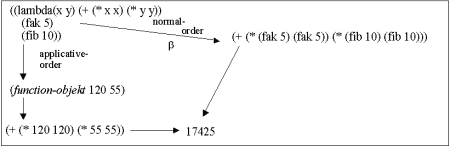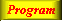Lecture 5 - Page 15 : 26
 Functional Programming in SchemeThe Order of Evaluation * Referential transparency Referential transparency An illustration of referential transparency * Introduction to evaluation order Arbitrary evaluation order - with some limits A motivating example A motivating example - clarification * Rewrite rules, reduction, and normal forms Rewrite rules The alpha rewrite rule The beta rewrite rule The eta rewrite rule Normal forms The ordering of reductions An example of normal versus applicative evaluation Theoretical results Practical implications Conditionals and sequential boolean operators Lazy evaluation * Delayed evaluation and infinite lists in Scheme Delayed evaluation in Scheme Examples of delayed evaluation Infinite lists in Scheme: Streams Example streams Stream example: The sieve of Eratosthenes Applications of The sieve of Eratosthenes
 An example of normal versus applicative evaluation
 Reduction of the expression ((lambda(x y) (+ (* x x) (* y y))) (fak 5) (fib 10))Normal vs. applicative reduction of a Scheme expressionThe necessary Scheme stuff to evaluate the expression.

 It appears to be the case that normal order reduction can lead to repeated evaluation of the same subexpression